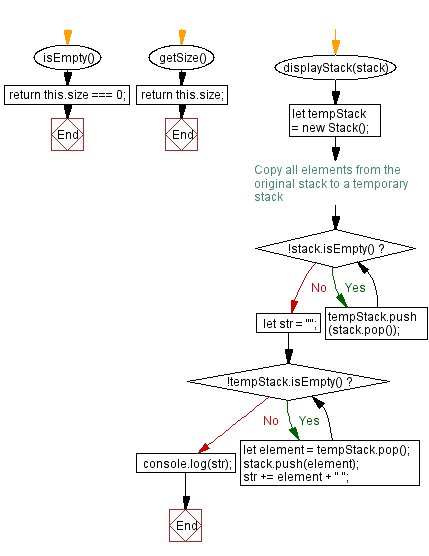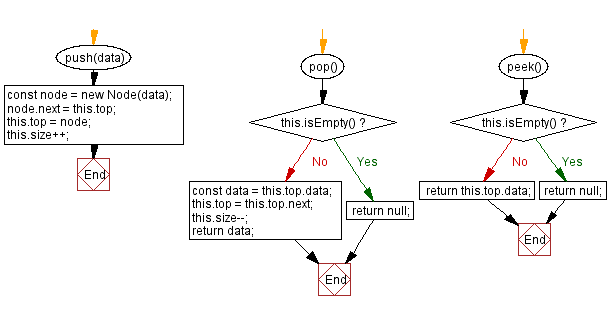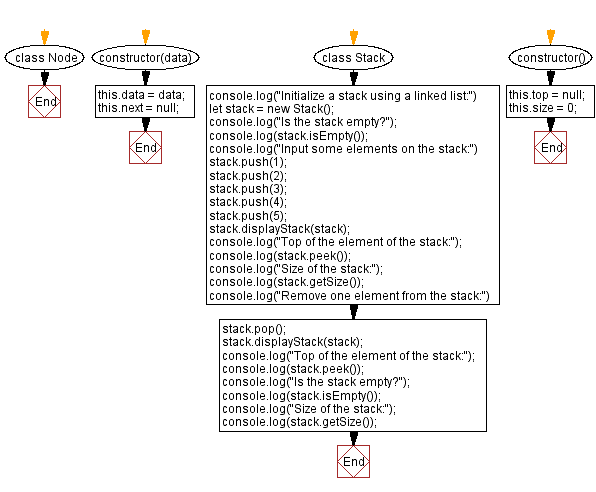# JavaScript Exercises: Implement a stack using a linked list

## JavaScript Stack: Exercise-21 with Solution

Write a JavaScript program to implement a stack using a linked list with push and pop operations. Find the top element of the stack and check if the stack is empty or not.

Sample Solution:

JavaScript Code:

``````class Node {
constructor(data) {
this.data = data;
this.next = null;
}
}

class Stack {
constructor() {
this.top = null;
this.size = 0;
}
push(data) {
const node = new Node(data);
node.next = this.top;
this.top = node;
this.size++;
}

pop() {
if (this.isEmpty()) {
return null;
}
const data = this.top.data;
this.top = this.top.next;
this.size--;
return data;
}

peek() {
if (this.isEmpty()) {
return null;
}
return this.top.data;
}

isEmpty() {
return this.size === 0;
}

getSize() {
return this.size;
}

displayStack(stack) {
let tempStack = new Stack();

// Copy all elements from the original stack to a temporary stack
while (!stack.isEmpty()) {
tempStack.push(stack.pop());
}
let str = "";
while (!tempStack.isEmpty()) {
let element = tempStack.pop();
stack.push(element);
str += element + " ";
}
console.log(str);
}

}

console.log("Initialize a stack using a linked list:")
let stack = new Stack();
console.log("Is the stack empty?");
console.log(stack.isEmpty());
console.log("Input some elements on the stack:")
stack.push(1);
stack.push(2);
stack.push(3);
stack.push(4);
stack.push(5);
stack.displayStack(stack);
console.log("Top of the element of the stack:");
console.log(stack.peek());
console.log("Size of the stack:");
console.log(stack.getSize());
console.log("Remove one element from the stack:")
stack.pop();
stack.displayStack(stack);
console.log("Top of the element of the stack:");
console.log(stack.peek());
console.log("Is the stack empty?");
console.log(stack.isEmpty());
console.log("Size of the stack:");
console.log(stack.getSize());
```
```

Sample Output:

```Initialize a stack using a linked list:
Is the stack empty?
true
Input some elements on the stack:
1 2 3 4 5
Top of the element of the stack:
5
Size of the stack:
5
Remove one element from the stack:
1 2 3 4
Top of the element of the stack:
4
Is the stack empty?
false
Size of the stack:
4
```

Flowchart:Live Demo:

See the Pen javascript-stack-exercise-20 by w3resource (@w3resource) on CodePen.

Improve this sample solution and post your code through Disqus

Stack Previous: Merge two stacks into one.
Stack Exercises Next: Convert a stack into an array.

What is the difficulty level of this exercise?

Test your Programming skills with w3resource's quiz.

﻿